Algebra 1 : How to write expressions and equations

Example Questions

Example Question #91 : How To Write Expressions And Equations

Express the product of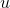and forty-nine.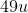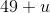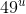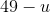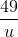Explanation:

Take every word and translate into math. Product indicates multiplication. So we multiplyandto get.

Example Question #92 : How To Write Expressions And Equations

Express quotient ofand six.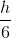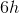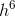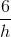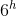Explanation:

Take every word and translate into math. Quotient indicates division. So we havelisted first being the numerator andlisted last being the denominator. Our answer is.

Example Question #93 : How To Write Expressions And Equations

Express the quotient of ten and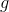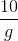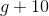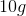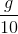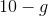Explanation:

Take every word and translate into math. Quotient indicates division. So we havelisted first being the numerator andlisted last being the denominator. Our answer is.

Example Question #92 : How To Write Expressions And Equations

Express five raised to thepower.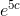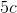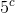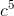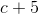Explanation:

Take every word and translate into math. Five raised to some power meansis the base andpower is the exponent. We haveas our answer.

Example Question #1011 : Linear Equations

Expressraised to the fourth power.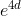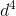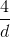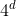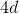Explanation:

Take every word and translate into math.raised to some power meansis the base andpower is the exponent. We haveas our answer.

Example Question #93 : How To Write Expressions And Equations

Express the sum ofandis forty.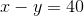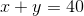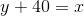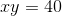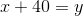Explanation:

Take every word and translate into math. Sum is addition. Is means equal sign. So we have.

Example Question #94 : How To Write Expressions And Equations

Express thirty-five is less than.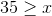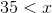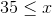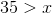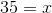Explanation:

Take every word and translate into math. Is less than means we need to use the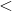symbol. We should haveExample Question #95 : How To Write Expressions And Equations

Express hundred is less than or equal to.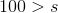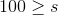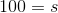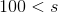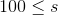Explanation:

Take every word and translate into math. Is less than or equal to means we need to use the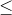symbol. We should haveExample Question #96 : How To Write Expressions And Equations

Express twelve is greater than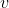.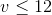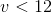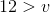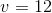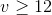Explanation:

Take every word and translate into math. Is greater than means we need to use the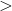symbol. We should haveExample Question #1016 : Linear Equations

Which equation represents the expression below?

Seven more than, a numbersquared multiplied by five.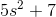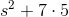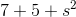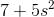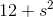Explanation:

Seven more than indicates that it comes afterwards, meaning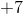at the end.

A numbersquared multiplied by five indicates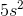.

It is written as